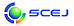English page## \$B^5-G09V1i(B

\$B:G=*99?7F|;~!'(B2014-08-08 13:21:03

### \$BM%=(O@J8>^(B

 \$BBh(B 1 \$BF|!&(BB \$B2q>l(B B123 \$B!V5\$1U3&LL\$N4J0WE*?tCM7W;;K!!W(B (16:20\$B!A(B16:40) (\$B=;M'2=3X(B) \$B%@!<%k(B \$B%"%S%J!<%V(B \$B;a(B\$B!&(B \$BEgED(B \$BD>\$B!&(B \$BIZ;3(B \$BL@CK(B \$B;a(B \$BBh(B 1 \$BF|!&(BC \$B2q>l(B C109 \$B!VBN@QJQ2=\$rH<\$&8G2=%W%m%;%97W;;\$N\$?\$a\$N%U%m%s%H%H%i%C%-%s%0K!!W(B (11:40\$B!A(B12:00) (\$BN)L?4[BgM}9)(B) Vu Truong \$B;a(B\$B!&(B (\$B%N!<%H%k%@%`Bg9)(B) Tryggvason Gretar \$B;a(B\$B!&(B (\$B:k6LBg1!(B) \$BK\4V(B \$B=S;J(B \$B;a(B\$B!&(B (\$BN)L?4[BgM}9)(B) Wells John \$B;a(B\$B!&(B (\$BN)L?4[M}9)(B) \$B9bAR(B \$B=(9T(B \$B;a(B \$BBh(B 2 \$BF|!&(BE \$B2q>l(B E204 \$B!VA4LVMe%_%k%/%Z%W%A%I%"%l%\$\$rMQ\$\$\$?%"%l%k%.! (10:00\$B!A(B10:20) (\$BL>Bg1!9)(B) \$BK\B?(B \$BM5G7(B \$B;a(B\$B!&(B (\$BL>Bg0e(B) \$BCf[=(B \$B>;90(B \$B;a(B\$B!&(B (\$BL>Bg1!9)(B) \$BJR;3(B \$B??(B \$B;a(B\$B!&(B (\$BL>Bg1!AOLt2J3X(B) \$B2CF#(B \$BN5;J(B \$B;a(B\$B!&(B (\$BL>Bg1!9)(B) \$BBg2OFb(B \$BH~F`(B \$B;a(B\$B!&(B (\$BL>BgM=KIAa4|0eNEAO@.%;(B) \$B5HED(B \$B0B;R(B \$B;a(B \$BBh(B 2 \$BF|!&(BJ \$B2q>l(B J201 \$B!V%P%\$%*%W%m%T%l%s9g@.H?1~%W%m%;%9\$N%7%_%e%l!<%?!<3+H/!W(B (9:00\$B!A(B9:20) (\$B5~Bg9)(B) \$BC+8}(B \$BCR(B \$B;a(B\$B!&(B (\$B;:Am8&(B) \$BBg?9(B \$BN4IW(B \$B;a(B\$B!&(B \$B2OB<(B \$B8wN4(B \$B;a(B\$B!&(B \$BJR2,(B \$B{D(B \$B;a(B\$B!&(B (\$BJ<8K8)Bg9)(B) \$B;3K\(B \$BBs;J(B \$B;a(B\$B!&(B (\$B;:Am8&(B) \$BCf4d(B \$B>!(B \$B;a(B\$B!&(B \$B9b66(B \$B8|(B \$B;a(B\$B!&(B \$BF#C+(B \$BCiGn(B \$B;a(B \$BBh(B 1 \$BF|!&(BN \$B2q>l(B N121 \$B!VIT2D5U@-\$H%(%/%;%k%.!<\$N4QE@\$+\$i\$N<+8JG.:F@8G.%W%m%;%9\$NI>2A!W(B (15:40\$B!A(B16:00) (\$BElBg@88&(B) \$B4Eht(B \$B\$B!&(B (\$BElBg1!9)(B) \$B>.C+(B \$BM#(B \$B;a(B\$B!&(B (\$BEl9)Bg1!9)(B) \$B%"%:%\$%C%:(B \$B%`%O%s%^%I(B \$B;a(B\$B!&(B (\$B?@8M@=9]=j(B) \$B4_K\(B \$B7<(B \$B;a(B\$B!&(B (\$BElBg@88&(B) \$BDi(B \$BFX;J(B \$B;a(B \$BBh(B 2 \$BF|!&(BN \$B2q>l(B N218 \$B!V2aK0OBHy:Y7k>=%9%i%j!<\$K\$h\$k(BLiBr\$B!&?e7O5[<}<0%R!<%H%]%s%W@-G=8~>e8z2L!W(B (14:40\$B!A(B15:00) (\$B4tIlBg9)(B) \$BHDC+(B \$B5A5*(B \$B;a(B\$B!&(B (\$BL>Bg9)(B) \$B;T66(B \$B?-5W(B \$B;a(B\$B!&(B (\$B4tIlBg9)(B) \$B>.NS(B \$B?.2p(B \$B;a(B\$B!&(B \$B4]LS(B \$B8,\$B!&(B \$BA}0f(B \$BN6Li(B \$B;a(B \$BBh(B 1 \$BF|!&(BO \$B2q>l(B O109 \$B!V@PC:%A%c! (11:40\$B!A(B12:00) (\$B4tIlBg9)(B) \$BHDC+(B \$B5A5*(B \$B;a(B\$B!&(B (\$BL>Bg1!9)(B) \$B9b:d(B \$BJ8:H(B \$B;a(B\$B!&(B (\$B4tIlBg9)(B) \$B>.NS(B \$B?.2p(B \$B;a(B \$BBh(B 1 \$BF|!&(BP \$B2q>l(B P113 \$B!V(BDevelopment of New Hybrid VOCs Treatment Process using Activated Carbon and Electrically Heated Alumite Catalyst\$B!W(B (13:00\$B!A(B13:20) (\$BG@9)Bg(B) \$B;38}(B \$B=SM:(B \$B;a(B\$B!&(B \$B55;3(B \$B=(M:(B \$B;a(B\$B!&(B \$B:y0f(B \$B@?(B \$B;a(B\$B!&(B \$B@DLZ(B \$BOBLi(B \$B;a(B \$BBh(B 1 \$BF|!&(BQ \$B2q>l(B Q113 \$B!V%7%j%+Kl\$K\$*\$1\$k6E=L@-5\$BN\$N%_%/%m9&= (13:00\$B!A(B13:20) (\$B9-Bg9)(B) \$B5H2,(B \$BJ~5W(B \$B;a(B\$B!&(B \$BD9_7(B \$B425,(B \$B;a(B\$B!&(B \$B6b;X(B \$B@58@(B \$B;a(B\$B!&(B \$BETN1(B \$BL-N;(B \$B;a(B

\$B9V1iH/I=%W%m%0%i%`(B
\$B2=3X9)3X2q(B \$BBh(B46\$B2s=)5(Bg2q(B(C) 2014 \$B8x1W
Most recent update: 2014-08-08 13:21:03
E-mail: inquiry-46fwww3.scej.org
This page was generated byeasp 2.34; proghtml 2.34 (C)1999-2014 kawase